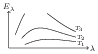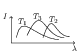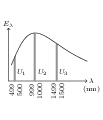# Wien's Displacement Law

## Problems from IIT JEE

Problem (IIT JEE 2005):Variation of radiant energy emitted by the sun, filament of tungsten lamp, and welding arc as a function of its wavelength is shown in figure. Which of the following option gives the correct match?

1. Sun-$T_1$, tungsten filament-$T_2$, welding arc-$T_3$
2. Sun-$T_2$, tungsten filament-$T_1$, welding arc-$T_3$
3. Sun-$T_3$, tungsten filament-$T_2$, welding arc-$T_1$
4. Sun-$T_1$, tungsten filament-$T_3$, welding arc-$T_2$

Solution: The Wien's displacement law is $\lambda_\text{max}T=b$. The knowledge of $\lambda_\text{max}$ or $T$ of the given sources will help in getting the answer. Typical temperature of tungsten filament in lamp is 3000 K. The surface temperature of the sun is 5800 K. The temperature of welding arc varies from 6000 K to 30000 K.

Problem (IIT JEE 2004): Three discs, $A$, $B$ and $C$ having radii 2 m, 4 m and 6 m, respectively are coated with carbon black on their outer surfaces. The wavelengths corresponding to maximum intensity are 300 nm, 400 nm and 500 nm, respectively. The power radiated by them are $Q_A$, $Q_B$ and $Q_C.$ Then,

1. $Q_A$ is maximum
2. $Q_B$ is maximum
3. $Q_C$ is maximum
4. $Q_A=Q_B=Q_C$

Solution: The temperature of a black body and the wavelength corresponding to its maximum intensity are related by the Wien's displacement law, \begin{align} \lambda_m T=b. \end{align} The power radiated by a black body at temperature $T$ having surface area $A$ and emissivity $e$ is given by the Stefan's law, \begin{align} Q=\sigma e A T^4. \end{align} For a thin disc of radius $r$ emitting from both surfaces, $A=2\pi r^2$. Eliminate $T$ from above equations and substitute for $A$ to get, \begin{align} Q=2\pi\sigma e b^4 \left( r^2/\lambda_m^4\right), \nonumber \end{align} which gives, \begin{align} Q_A:Q_B:Q_C=\frac{r_A^2}{\lambda_{A}^4}:\frac{r_B^2}{\lambda_{B}^4}:\frac{r_C^2}{\lambda_{m}^4}=0.049:0.0625:0.057. \nonumber \end{align}

Problem (IIT JEE 2000):The plots of intensity versus wavelength for three black bodies at temperatures $T_1$, $T_2$ and $T_3,$ respectively are as shown in figure. Their temperatures are such that,

1. $T_1 > T_2 > T_3$
2. $T_1 > T_3 > T_2$
3. $T_2 > T_3 > T_1$
4. $T_3 > T_2 > T_1$

Solution: The Wien's displacement law relates wavelength at which intensity attains maximum to the temperature of the body, $\lambda_m T=b$. In given cases, $\lambda_{m,1} < \lambda_{m,3} < \lambda_{m,2}$ and hence $T_1 > T_3 > T_2$.

Problem (IIT JEE 1998) A black body is at a temperature of 2880 K. The energy of radiation emitted by this body with wavelength between 499 nm and 500 nm is $U_1$, between 999 nm and 1000 nm is $U_2$ and between 1499 nm and 1500 nm is $U_3$. The Wien constant, $b=2.88\times{10}^{6}\;\mathrm{nm K}$. Then,

1. $U_1=0$
2. $U_3=0$
3. $U_1>U_2$
4. $U_2>U_1$

Solution:The Wien's displacement law gives, \begin{align} \lambda_m=\frac{b}{T}=\frac{2.88\times{10}^{6}}{2880}=1000\;\mathrm{nm}. \nonumber \end{align} Thus radiated energy $E_\lambda$ attains its maximum at $\lambda_m=1000\;\mathrm{nm}$. The variation of $E_\lambda$ with $\lambda$ is shown in figure. The area under the curve for given wavelength range gives radiated energy in that wavelength range i.e., $U_\lambda=E_\lambda\Delta\lambda$. The figure clearly shows that $U_1 < U_3 < U_2$.

Problem (IIT JEE 1997): The intensity of radiation emitted by the sun has its maximum value at a wavelength of 510 nm and that emitted by the north star has the maximum value at 350 nm. If these stars behaves like black bodies, then the ratio of the surface temperature of the sun and the north star is,

1. $1.46$
2. $0.69$
3. $1.21$
4. $0.83$

Solution: The Wien's displacement law relates wavelength at which intensity attains maximum to the temperature of the body, $\lambda_m T=b$. Thus, \begin{align} \frac{T_\text{sun}}{T_\text{star}}=\frac{\lambda_\text{m,star}}{\lambda_\text{m,sun}}=\frac{350}{510}=0.69. \nonumber \end{align}